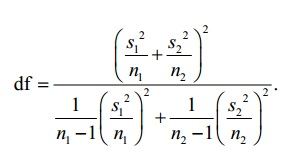# Satterthwaite Formula for Degrees of Freedom

Share on

Probability and Statistics > TI-83 Calculator > Satterthwaite Formula

## What is the Satterthwaite Formula for Degrees of Freedom??

The Satterthwaite approximation is a formula used in a two-sample t-test for degrees of freedom. It’s used to estimate an “effective degrees of freedom” for a probability distribution formed from several independent normal distributions where only estimates of the variance are known. It was originally developed by statistician Franklin E. Satterthwaite.

It’s a formula used in AP Statistics. You don’t really need to use the formula, as the TI-83 calculator can calculate the degrees of freedom for you. Or, you could be conservative and calculate the degrees of freedom by using the smaller of n1 – 1 or n2 – 1. The Satterthwaite formula is:In order to understand the Satterthwaite formula you should first be familiar with a chi-square distribution and a t-distribution. Your AP Statistics teacher may work through the formula with you. It’s possibly the ugliest, most daunting equation you’ll come across in the class. You can find the rather complicated mathematics behind it here.

But the good news! You’ll be using the TI-83 calculator on the exam. Therefore it’s completely unnecessary that you know how to work the formula by hand. You just need to know how to use the tcdf function on your calculator.

## Satterthwaite formula for a two-sample t-test: Steps

Sample question: Find P(X> = 1.645) with 11 degrees of freedom for a two-sample t-test.
Step 1:Press the 2nd key, then press VARS for DISTR.

Step 2:Press 5 for t-distribution (tcdf().

Step 3:Enter the parameters for the distribution on the screen. Type 1.645 then a comma(,) then 1 2nd comma(,) (to read EE) 99 (to read 1E99) then comma(,) 11) (for right parenthesis) then ENTER.